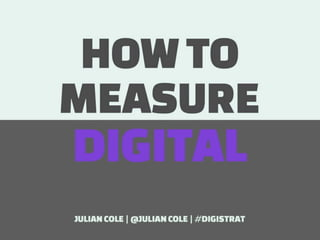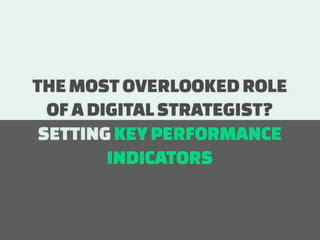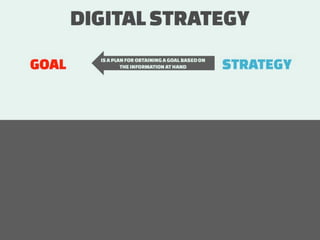Successfully reported this slideshow.×
1 of 71

How to measure Digital

293

Share

How to measure Digital looks at the important role of Key Performance Indicators and how you create them. It takes a Communication Objective and turns it into Strategy that leads to Tactics to support the Strategy. The final piece is putting in place Key Performance Indicators which makes up the parts of the Communications Objective.

See all

See all

• \n
• \n
• \n
• \n
• \n
• \n
• \n
• \n
• \n
• \n
• \n
• \n
• \n
• \n
• \n
• \n
• \n
• \n
• \n
• \n
• \n
• \n
• \n
• \n
• \n
• \n
• \n
• \n
• \n
• \n
• \n
• \n
• \n
• \n
• \n
• \n
• \n
• \n
• \n
• \n
• \n
• \n
• \n
• \n
• \n
• \n
• \n
• \n
• \n
• \n
• \n
• \n
• \n
• \n
• \n
• \n
• \n
• \n
• \n
• \n
• \n
• \n
• \n
• \n
• \n
• \n
• \n
• \n
• \n
• \n
• \n